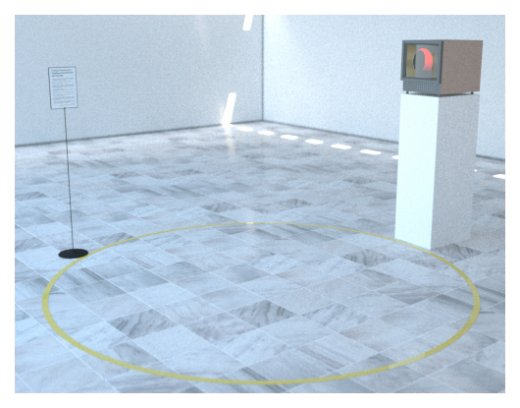### temporary border of the antipode's expansionist republic

Exhibition "Distances"
Spring 2010 in Paris
Summer-Fall 2010 Taiwan

A circle is the set of all the point that are at the same distance (the radius) of a point (the centre). (doc. 1)

Let's call "inside" the set of points which distance to this point is inferior, and by extension "outside" those with a greater distance. (doc. 2)

Now let our circle's radius grow on the surface of the earth. (doc. 3)
When the radius is equal to half the meridian, the perimeter of the circle is equal to the perimeter of the equator. When it goes bigger, the perimeter tends to go smaller.
When the radius approaches the length of the meridian, we get a small circle.

This installation displays a circle on the floor, but it's centre isn't where it seems to be, it's at the antipodal point. (doc. 4)

Remarks :
— The inside is "outside" the circle.
— The outside is "inside" the circle.
— the radius of the circle is equal to the lenght of the meridian minus the radius of the apparent circle.doc. 1doc. 2doc. 3doc. 4